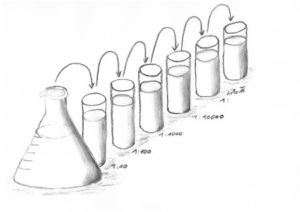bartelt | Dilution series

# Dilution series

### Calculate your arithmetic or geometric dilution series

Dilution results in various dilution steps or solution concentrations that differ, for example, in the concentration of the material quantities.

Serial dilution:

In the case of serial dilution (also known as continuous or geometrical dilution), the last dilution step is used as the starting solution for the subsequent dilution step.

Therefore, it involves the repeated dilution of a solution with the purpose of geometrically diluting the original solution.Parallel dilution:

In this case, all steps of the dilution are carried out directly from the stock solution.
This procedure involves the arithmetic dilution of the starting solution.

Measure values:

You can now measure from the individual diluted positions. If there is a measured value (dilution sufficient), this can be entered for this position.

If several measured values ​​are entered, an average value is calculated automatically, using the dilution factor.

### Calculation

$\frac{1000*\mathrm{measured value}*\mathrm{dilution factor}}{\mathrm{target volume}}=\mathrm{Result in unit/l}$